# Axis Of Symmetry For A Parabola (10 Key Things To Know)

The axis of symmetry is a line that splits an object in half to give us “mirror images” on both sides of the line.  For a parabola, the axis of symmetry is determined by two coefficients of a quadratic function.

So, what do you need to know about a parabola’s axis of symmetry?  A parabola’s axis of symmetry is a vertical line through the vertex. The quadratic function f(x) = ax2 + bx + c has axis of symmetry x = -b / 2a (a vertical line). The x-value of the axis of symmetry is the average of the two zeros of the corresponding quadratic function f(x) = ax2 + bx + c.

Of course, the x-value of the axis of symmetry can be positive, negative, or zero.  Every parabola has a single axis of symmetry, which is independent of the value of c.

In this article, we’ll talk about the axis of symmetry of a parabola and what it means.  We’ll also look at how to find the axis of symmetry and answer some common questions about the topic.

Let’s get started.

## What Is The Axis Of Symmetry Of A Parabola?

The axis of symmetry of a parabola is a vertical line through the vertex (turning point) of the parabola.  For the quadratic function f(x) = ax2 + bx + c, the axis of symmetry is the vertical line x = -b / 2a.

The x-value of the axis of symmetry is the average of the two zeros of the quadratic.  We can see this by adding the two zeros from the quadratic formula (the radical terms will cancel) and dividing by 2.

### How To Find Axis Of Symmetry For A Parabola

If you know the quadratic formula that corresponds to the parabola, use the following formula for the axis of symmetry:

• x = -b / 2a

If you do not know the equation of the corresponding quadratic, you can take the average of the zeros of the equation if you know them.

If you do not know the zeros of the quadratic equation, look at the graph to find them.  If you cannot determine the zeros (or if the parabola has none for a negative discriminant), then look for the extreme point of the graph (vertex).

The x-coordinate of the maximum value (for a concave parabola) or the minimum value (for a convex parabola) will tell you the axis of symmetry.

#### Example: Finding The Axis Of Symmetry

Consider the quadratic function f(x) = 3x2 + 24x + 7.  We have coefficients of a = 3, b = 24, and c = 7.

Using the formula for the axis of symmetry, we get:

• x = -b / 2a
• x = -24 / 2(3)
• x = -4

So the axis of symmetry is the vertical line x = -4.  We can see this in the graph below.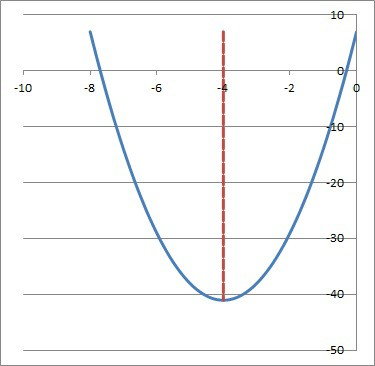The parabola from the quadratic function f(x) = 3x2 + 24x + 7 (blue curve). The axis of symmetry is the vertical line x = -4 (dashed red line).

### Does A Parabola Always Have An Axis Of Symmetry?

By the definition of a parabola, it will always have an axis of symmetry.  A parabola is simply the graph of a quadratic function f(x) = ax2 + bx + c.

The axis of symmetry will always be the vertical line x = -b / 2a.  Note that the axis of symmetry does not depend on the value of c, since this constant value simply shifts a parabola up or down.

For example, the parabola from f(x) = 2x2 + 12x has axis of symmetry x = -b / 2a = -12 / 2(2) = -3.  Similarly, the parabola from g(x) = 2x2 + 12x + 5 has the same axis of symmetry (x = -3) since it is the same graph shifted up 5 units.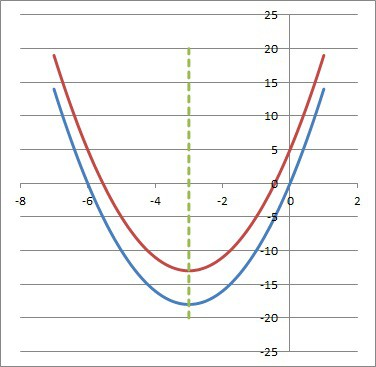The parabola from the quadratic function f(x) = 2x2 + 12x (blue curve) and g(x) = 2x2 + 12x + 5 (red curve). The axis of symmetry for both parabolas is the vertical line x = -3 (dashed green line).

### Is Axis Of Symmetry The Same As Line Of Symmetry?

The axis of symmetry for a parabola is the same as the line of symmetry for a parabola.  Both refer to the vertical line x = -b / 2a that goes through the vertex of the parabola.

### Is Axis Of Symmetry The Same As Vertex (Turning Point)?

The axis of symmetry is not exactly the same as the vertex (or turning point) of a parabola, since one is a line and one is a point.  However, the two concepts are related.  Remember that:

• The vertex is an extreme point on the parabola with x-coordinate b / 2a.  For a concave (concave down) parabola, the vertex is the maximum.  For a convex (concave up) parabola, the vertex is the minimum.
• The axis of symmetry is the vertical line x = -b / 2a that goes through the vertex of the parabola.  This line cuts the parabola into two equal halves (left and right), which are mirror images of one another.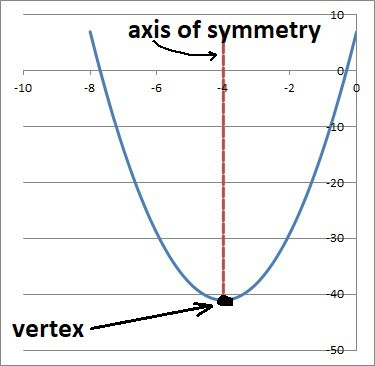The parabola from the quadratic function f(x) = 3x2 + 24x + 7 (blue curve). The axis of symmetry is the vertical line x = -4 (dashed red line), which goes through the vertex, which is the point (-4, -41) at the bottom of the curve.

### Is Axis Of Symmetry The Same As The X Intercept?

The axis of symmetry is not the same as the x-intercept for a parabola.  Remember that:

• The x-intercept is a point where the parabola crosses the x-axis (that is, where y = 0).  A parabola may have 2, 1, or 0 x-intercepts (this depends on the sign of the discriminant: positive, zero, or negative – you can learn more about what the discriminant tells you in my article here).
• The axis of symmetry is the vertical line x = -b / 2a that goes through the vertex of the parabola.  The axis of symmetry will only go through the x-intercept when the parabola has a single x-intercept at the vertex (this happens when the discriminant is zero, or b2 – 4ac = 0).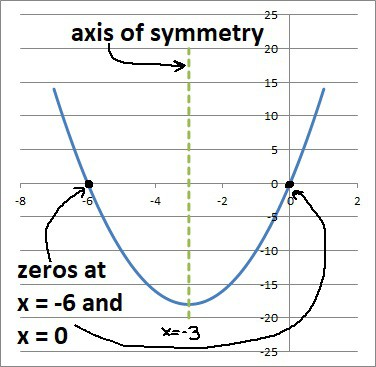The parabola from the quadratic function f(x) = 2x2 + 12x (blue curve). The axis of symmetry is the vertical line x = -3 (vertical line), which does not intersect the zeros (x = -6 and x = 0).

### Can The Axis Of Symmetry Be Zero?

The axis of symmetry can be zero (that is, the vertical line x = 0).  We can find out exactly when this happens by using the formula for the line of symmetry:

• Line of symmetry = zero
• -b / 2a = 0
• -b = 0
• b = 0

So, the axis of symmetry is zero (the vertical line x = 0) when b = 0.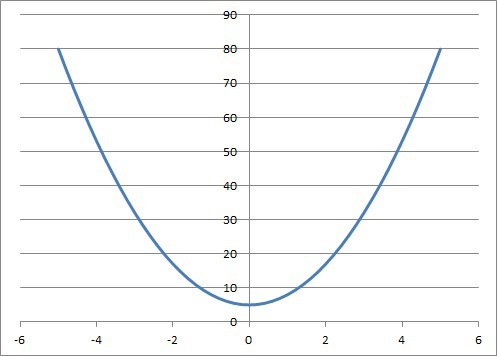The parabola from the quadratic function f(x) = 3x2 + 5 has its axis of symmetry at x = 0, since b = 0 in the quadratic equation.

This means that the corresponding quadratic function will have the form:

• f(x) = ax2 + bx + c
• f(x) = ax2 + (0)x + c
• f(x) = ax2 + c

So in this case, the quadratic function is a quadratic binomial.  The zeros will be x = +√(-c/a) and x = -√(-c/a) (note that these will be imaginary numbers if a and c have the same sign).

### Can The Axis Of Symmetry Be Negative?

The axis of symmetry can be negative (that is, the line x = d for a value d < 0).  We can find out exactly when this happens by using the formula for the line of symmetry:

The left side of this inequality will be positive when a and b have the same sign, and negative when they have opposite signs (it will be zero if b is zero).

So, the axis of symmetry will be negative whenever a and b have the same sign (both positive or both negative).

### Can The Axis Of Symmetry Be A Fraction?

The axis of symmetry can be a fraction.  We can find out exactly when this happens by using the formula for the line of symmetry:

• Line of symmetry is a fraction
• -b / 2a is a fraction

This will happen in the following cases (not an exhaustive list):

• when b is an odd integer (since it won’t have a factor of 2 to cancel the 2 in the denominator).
• when b is even, but not a multiple of a (since b / a will not reduce to an integer).

For example, the quadratic function f(x) = 3x2 + 2x + 1 is a parabola with axis of symmetry x = -b / 2a = -2 / 2(3) = -1/3, which is a fraction.  The value of b is even, but it is not a multiple of a (2 is not a multiple of 3).

### Can The Axis Of Symmetry Be A Y Value (Horizontal)?

The axis of symmetry can be a y value (a horizontal line) in the case of a “sideways” parabola of the form x = ay2 + by + c.  However, this will not be a function in the usual sense, since it fails the vertical line test.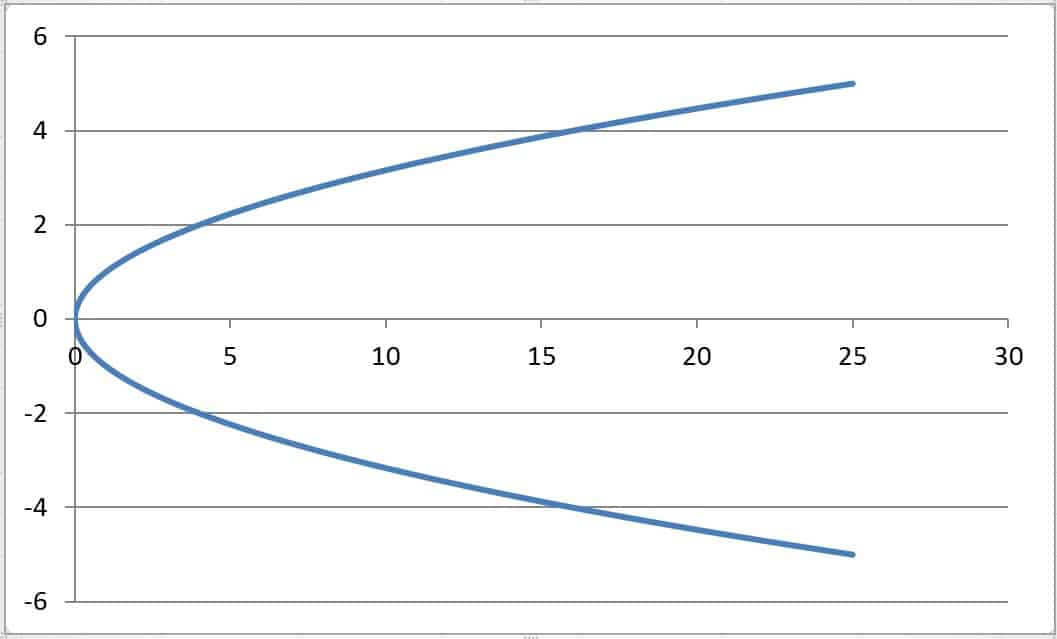A “sideways” parabola of the form x = ay2 + by + c has a horizontal axis of symmetry (here, the line y = 0). This is not a function, since it fails the vertical line test.

However, we can always just switch the variables x and y to view the parabola in the usual way that we are familiar with.

## Conclusion

Now you know about the axis of symmetry for a parabola and what it means.  You also know how to find the axis of symmetry and the forms it can take.

You can learn about the focus of a parabola and what it means here.

You can learn how to find the domain and range of a parabola here.

You can learn about the related concept of hyperbola (and how to graph them) here.